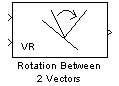# Rotation Between 2 Vectors

Virtual world rotation between two 3-D vectors

• Library:
• Simulink 3D Animation / Utilities

•## Description

The Rotation Between 2 Vectors takes the input of two 3-by-1 vectors and returns a virtual world rotation (specified as a 4-element vector defining the axis and angle) that is needed to transform the first input vector to the second input vector.

## Ports

### Input

expand all

The input signal is a 3-element vector whose elements correspond to its magnitudes along the $\stackrel{^}{i},\stackrel{^}{j},\stackrel{^}{k}$ unit vectors, respectively.

Data Types: `double`

The input signal is a 3- element vector whose elements correspond to its magnitudes along the $\stackrel{^}{i},\stackrel{^}{j},\stackrel{^}{k}$ unit vectors, respectively.

Data Types: `double`

### Output

expand all

The output of the block is an axis-angle representation of the rotation needed to transform the first input vector to the second input vector.

Data Types: `double`

## Version History

Introduced in R2006a